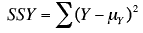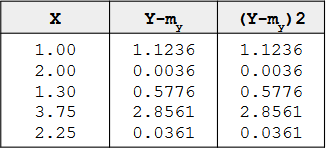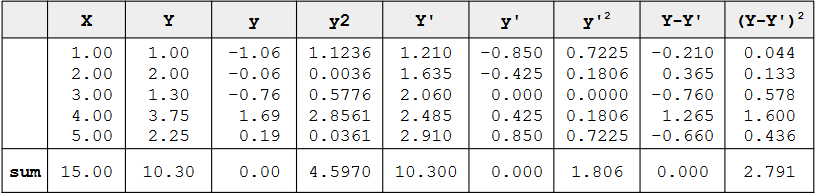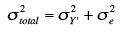# Partitioning the Sums of Squares

One useful aspect of regression is that it can divide the variation in Y into two parts:

• the variation of the predicted scores
• the variation in the errors of prediction

# The variation of Y

• the sum of squares Y
• defined as the sum of the squared deviations of Y from the mean of Y

## Formula of the Variation of Y

In the population, the formula of The variation of Y

```where SSY is the sum of squares Y and
Y is an individual value of Y, and my is the mean of Y
```

## Example

The mean of Y is 2.06 and SSY is the sum of the values in third column and is equal to 4.597When computed in a sample, you should use the sample mean, M, in place of the population mean.

## Example• The column Y' were computed according to this equation.
• The column y' contains deviations of Y' from the mean Y'
• The column y'2 is the square of this column.
• The column Y-Y' contains the actual scores (Y) minus the predicted scores (Y')
• The column (Y-Y')2 contains the squares of these errors of prediction

# Sum of the squared deviations from the mean

SSY is the sum of the squared deviations from the mean.

• It is therefore the sum of the y2 column and is equal to 4.597.
• SSY can be partitioned into two parts:
1. the sum of squares predicted (SSY')
• The sum of squares predicted is the sum of the squared deviations of the predicted scores from the mean predicted score.
• In other words, it is the sum of the y'2 column and is equal to 1.806
2.the sum of squares error (SSE)
• The sum of squares error is the sum of the squared errors of prediction.
• It is there fore the sum of the (Y-Y')2 column and is equal to 2.791.
• This can be summed up as:
```SSY = SSY' + SSE
4.597 = 1.806 + 2.791
```

## Example

The sum of y and the sum of y' are both zero
This will always be the case because these variables were created by subtracting their respective means from each value.
The mean of Y-Y' is 0
This indicates that although some Y's are higher than there respective Y's and some are lower, the average difference is zero.
```SSY is the total variation
SSY' is the variation explained
SSE is the variation unexplained
```

Therefore, the proportion of variation explained can be computed as:

```Proportion explained = SSY'/SSY
```

Similarly, the proportion not explained is:

```Proportion not explained = SSE/SSY
```

## r2

There is an important relationship between the proportion of variation explained and Pearson's correlation:

r2 is the proportion of variation explained

Therefore,

• if r = 1, then the proportion of variation explained is 1
• if r = 0, then the proportion explained is 0;
• if r = 0.4, then the proportion of variation explained is 0.16

Since the variance is computed by dividing the variation by N (for a population) or N-1 (for a sample), the relationships spelled out above in terms of variation also hold for variance

### Example• the first term is the variance total
• the second term is the variance of Y'
• the last term is the variance of the errors of prediction (Y-Y')

Similarly, r2 is the proportion of variance explained as well as the proportion of variation explained.

# Summary Table

It is often convenient to summarize the partitioning of the data in a table.

• The degrees of freedom column (df) shows the degrees of freedom for each source of variation.
• The degrees of freedom for the sum of squares explained is equal to the number of predictor variables.
• This will always be 1 in simple regression.
• The error degrees of freedom is equal to the total number of observations minus 2.
• In this example, it is 5 - 2 = 3.
• The total degrees of freedom is the total number of observations minus 1.
Source Sum of Squares df Mean Square
Explained 1.806 1 1.806
Error 2.791 3 0.930
Total 4.597 4

# Quiz

1

If these data are converted to deviation scores, the last value (15) would have a value of

```Y
2
9
11
13
15
```

15

To compute a deviation score you subtract the mean. 15 - 10 is 5.

2

Compute the sum of squares Y.

```Y
2
9
11
13
15
```

100

To compute SSY, first compute the deviation scores (y) by subtracting the mean (10) from each number. Then square these values and add them together: (-8)2 + (-1)2 + 12 + 32 + 52 equals to 100

3

If SSY is 25.5 and SSY' is 18.3, what is SSE?

```Y
2
9
11
13
15
```

7.2

SSY is SSY' + SSE; SSE is SSY - SSY' 25.5 - 18.3 equals to 7.2

4

The larger ________ is, the larger the proportion of variation explained is.

 SSY SSY' SSE Y

False

Proportion of variation explained is SSY'/SSY, so as SSY' increases, so does the proportion of variation explained.

5

The proportion of variation explained is 0.3. If SSY is 20, what is SSY'?

6

Proportion explained is SSY'/SSY; SSY' is (.3)(20) equals to 6

6

If r is .84, what proportion of variation is explained?